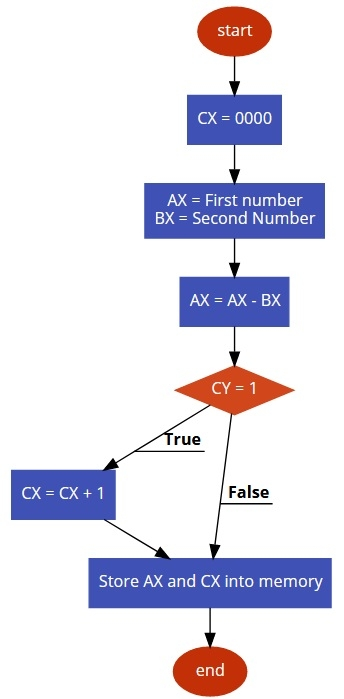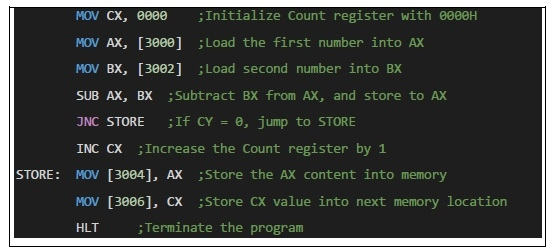# 8086 program to subtract two 16-bit numbers with or without borrow

In this program we will see how to subtract two 16-bit numbers with and without borrow.

## Problem Statement

Write 8086 Assembly language program to subtract two 16-bit number stored in memory location 3000H – 3001H and 3002H – 3003H.

## Discussion

8086 is 16-bit register. We can simply take the numbers from memory to AX and BX register, then subtract them using SUB instruction. When the Borrow is present, the CY flag will be 1, so we can store borrow into memory, otherwise only store AX into memory.

Data

3000
2D
3001
FE
3002
3003
BC

## Flow Diagram## Program